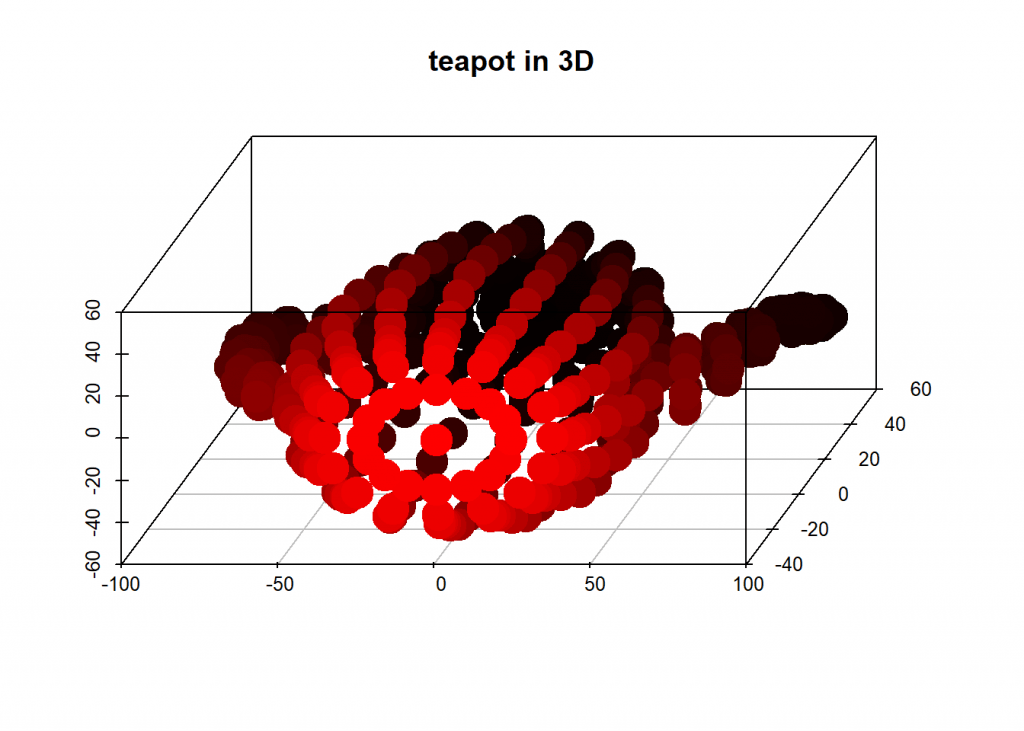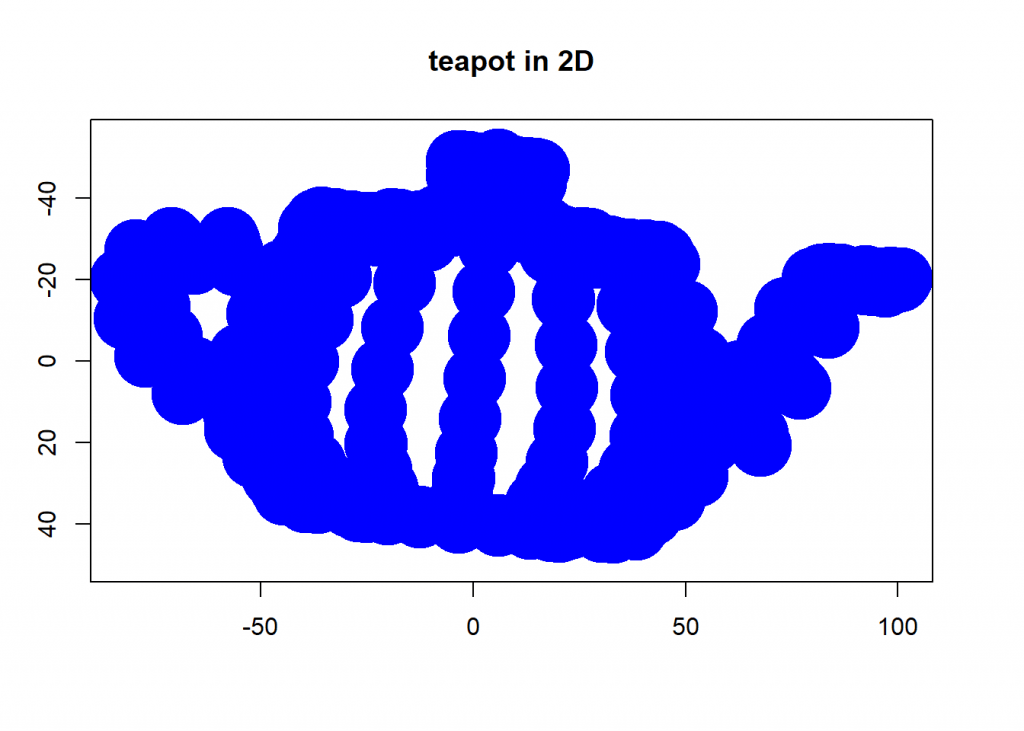Want to share your content on R-bloggers? click here if you have a blog, or here if you don't.

Principal component analysis (PCA) is a dimension-reduction method that can be used to reduce a large set of (often correlated) variables into a smaller set of (uncorrelated) variables, called principal components, which still contain most of the information.

PCA is a concept that is traditionally hard to grasp so instead of giving you the n’th mathematical derivation I will provide you with some intuition.

Basically PCA is nothing else but a projection of some higher dimensional object into a lower dimension. What sounds complicated is really something we encounter every day: when we watch TV we see a 2D-projection of 3D-objects!

Have a look at this instructive youtube video.

So what we want is not only a projection but a projection which retains as much information as possible. A good strategy is to find the longest axis of the object and after that turn the object around this axis so that we again find the second longest axis.

Mathematically this can be done by calculating the so called eigenvectors of the covariance matrix, after that we use just use the n eigenvectors with the biggest eigenvalues to project the object into n-dimensional space (in our example n = 2) – but as I said I won’t go into the mathematical details here (many good explanations can be found here on stackexchange).

To make matters concrete we will now recreate the example from the video above! The teapot data is from https://www.khanacademy.org/computer-programming/3d-teapot/971436783 – there you can also turn it around interactively.

Have a look at the following code:

```library(scatterplot3d)

dat <- matrix(unlist(teapot), ncol = 3, byrow = TRUE)
##      [,1] [,2] [,3]
## [1,] 40.6 28.3 -1.1
## [2,] 40.1 30.4 -1.1
## [3,] 40.7 31.1 -1.1
## [4,] 42.0 30.4 -1.1
## [5,] 43.5 28.3 -1.1
## [6,] 37.5 28.3 14.5

# teapot in 3D
scatterplot3d(dat[ , 1], dat[ , 2], dat[ , 3], highlight.3d = TRUE, angle = 75, pch = 19, lwd = 15, xlab = "", ylab = "", zlab = "", main = "teapot in 3D")
```And now we project that teapot from 3D-space onto a 2D-plane while retaining as much information as possible by taking the two eigenvectors with biggest eigenvalues:

```# PCA
(eigenval <- eigen(cov(dat))\$values)
##  2050.6935  747.5764  553.4070

eigenvec <- eigen(cov(dat))\$vectors
# take only the two eigenvectors with biggest eigenvalues
PCA_2 <- dat %*% eigenvec[ , 1:2]
plot(PCA_2, ylim = c(50, -55), pch = 19, lwd = 35, col= "blue", xlab = "", ylab = "", main = "teapot in 2D")
``````round(cumsum(eigenval)/sum(eigenval) * 100, 2) # cumulative percentage of retrieved information
##   61.18  83.49 100.00
```

That was fun, wasn’t it!

In data science you will often use PCA to condense large datasets into smaller datasets while retaining most of the information. PCA is like clustering an unsupervised learning method.